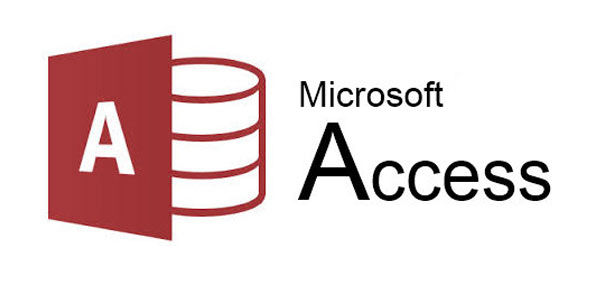# Microsoft Access Database Application Quiz Questions

30 Questions | Total Attempts: 1460SettingsRelated Topics
• 1.
Which of he following is NOT a part of a database?
• A.

Tables

• B.

Records

• C.

Fields

• D.

Worksheets

• 2.
In a database, each _________________ contains a collection of related data.
• A.

Table

• B.

Record

• C.

Field

• D.

None of the above

• 3.
In a database, a _____________ is a unit of information about one individual or item.
• A.

Table

• B.

Record

• C.

Field

• D.

File

• 4.
In a record, each separate piece of data, such as a last name, a first name, or an address, is called a _____________.
• A.

File

• B.

Field

• C.

Record

• D.

Table

• 5.
Databases are useful only if they are ___________.
• A.

Big

• B.

Detailed

• C.

Accurate

• D.

Expensive

• 6.
What does GIGO stand for?
• A.

Get in, get out

• B.

Garbage in, garbage out

• C.

Good information in, good information out

• D.

Garbage input, garbage output

• 7.
When creating the Mystery database, what database type did you create?
• A.

Relational database

• B.

Key field database

• C.

Organizational database

• D.

Flat file database

• 8.
The use of a password can help presere a database's _____________.
• A.

Privacy

• B.

Security

• C.

Accurace

• D.

Integrity

• 9.
Which of the following is a way to sort data?
• A.

Chronologically

• B.

Alphabetically

• C.

Numerically

• D.

All of the above

• E.

None of the above

• 10.
If you sort data in order of decreasing value such as Z-A, or 10-1, what type of sort are you using?
• A.

Ascending

• B.

Descending

• C.

Incremental

• D.

Exponential

• 11.
What is a query?
• A.

A question you ask a database

• B.

A question you ask a record

• C.

A question you ask a table

• D.

A question you ask a field

• 12.
What is WYSIWYG?
• A.

What you say is what you get

• B.

Why you say is why you get

• C.

What you state is what you've got

• D.

What you see is what you get

• 13.
What does SQL stand for?
• A.

Structured query language

• B.

Stated question language

• C.

System query lingo

• D.

Status query language

• 14.
What does a filter do?
• A.

Make more records available

• B.

Hide records from view

• C.

Slow down processing

• D.

Add to the quantity of data

• 15.
When I add my name to a store database, that is an example of ______________?
• A.

Batch processing

• B.

Database processing

• C.

Unit processing

• D.

Transactional processing

• 16.
A database is an organized collection of information.
• A.

True

• B.

False

• 17.
Databases typically store their data in one big table.
• A.

True

• B.

False

• 18.
A field's data type determines what kind of information can be stored there.
• A.

True

• B.

False

• 19.
A computerized database can store millions of telephone numbers.
• A.

True

• B.

False

• 20.
One disadvantage of computerized databases is that they limit your ability to arrange information.
• A.

True

• B.

False

• 21.
A database is a collection of related information, but a database management system is a type of software program.
• A.

True

• B.

False

• 22.
A multimedia database acts as an index to let users find and view all kinds of multimedia files.
• A.

True

• B.

False

• 23.
A query lets you speed up the prowsing process by finding information that matches specifically to your criteria.
• A.

True

• B.

False

• 24.
The same features that make databases efficient tools also enables them to keep data secure.
• A.

True

• B.

False

• 25.
If you sort a database's information chronologically, then you are sorting letters and symbols.
• A.

True

• B.

False

• 26.
Please give an example of the following data types. (each correct answer is worth 5 points):  A sample of the anwer is "Date/Time, is used to indicate date and time." 1.Text -
• 27.
Please give an example of the following data types. (each correct answer is worth 5 points):  A sample of the anwer is "Date/Time, is used to indicate date and time." 1.Autonumber -
• 28.
Please give an example of the following data types. (each correct answer is worth 5 points):  A sample of the anwer is "Date/Time, is used to indicate date and time." 1.Logical -
• 29.
Please give an example of the following data types. (each correct answer is worth 5 points):  A sample of the anwer is "Date/Time, is used to indicate date and time." 1.Memo -
• 30.
Please give an example of the following data types. (each correct answer is worth 5 points):  A sample of the anwer is "Date/Time, is used to indicate date and time." 1.Hyperlink -